# HBSE 6th Class Maths Solutions Chapter 6 Integers InText Questions

Haryana State Board HBSE 6th Class Maths Solutions Chapter 6 Integers InText Questions and Answers.

## Haryana Board 6th Class Maths Solutions Chapter 6 Integers InText Questions

Try These (Page 148)

Question 1.
Write the following numbers with appropriate signs :
(a) 100 m below sea level (b) 25°C above 0° temperature
(c) 15°C below 0° temperature (d) 5 number less than 0.
Solution:
(a) +100 m, (b) +25°C, (c) -15°C, (d) -5.

Try These (Page 151)

Question 1.
Mark -3, 7, -4, -8,1 and -5 on the number line. Can you tell which is the largest integer amongst these integers ?
Solution: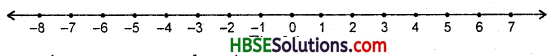7 is the largest integer amongst these integers.

Try These (Page 152)

Question 1.
Compare the following pairs of numbers using > or < :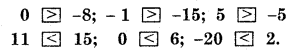From the above exercise, we arrived at the following conclusions, give examples of each :
(a) Every positive integer is larger than every negative integer.
e.g. 3 > – 1, 5 > -2, 6 > -3 etc.
(b) Zero is less than every positive integer.
e.g. 0 < 2, 0 < 5, 0 < 10 etc.
(c) Zero is larger than every negative integer.
e.g. 0 > -3, 0 > -7, 0 > -15 etc.
(d) Zero is neither a negative integer nor a positive integer.
(e) Farther a number from zero on the right, larger is its value.
e.g. 7 > 5, 8 > 3, 10 > 2 etc.
(f) Farther a number from zero on the left, smaller is its value.
e.g. -5 < -3, -7. < -4, -10 < -6 etc.

Try These (Page 159)

Question 1.
(a) (-11)+ (-12)
(b) (+10) + (+4)
(c) (-32)+ (-25)
(d) (+23) + (+40).
Solution:
(a) (-11) + (-12) = -23
(b) (+10) + (+4) =+14
(c) (-32) + (-25) = -57
(d) (+23) + (+40) = +63.

Try These (Page 160)

Question 1.
Find the solution of the following :
(a) (-7) + (+8)
(b) (-9) + (+13)
(c) (+7) + (-10)
(d) (+12) + (-7).
Solution:
(a) (-7) + (+8) = +1
(b) (-9) + (+13) = +4
(c) (+7) + (-10) = -3
(d) (+12) + (-7) = +5.

Try These (Page 162)

Question 1.
Find the solution of the following additions using a number line :
(a) (-2) + 6
(b) (-6) + 2
Make two such questions and solve them using the number line.
Solution:
(a)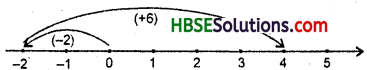First we move 2 steps to the left of 0 reaching -2 and then from this point we move 6 steps to the right. We reach the point 4. Thus, (-2) + (+6) = 4.

(b)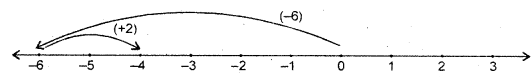First we move 6 steps to the left of 0 reaching -6 and then from this point we move 2 steps to the right. We reach the point -4. Thus, (-6) + (+2) = -4.

Question 2.
Find the solution of the following additions using a number line :
(i) (-4) + (+3)
(ii) (+4) + (-3)
Solution:
(i)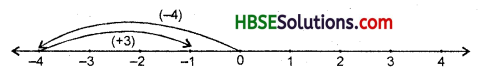First we move 4 steps to the right of 0 reaching 4 and then from this point we move 3 steps to the right. We reach the point -1. Thus, (-4) + (+3) = -1.

(ii)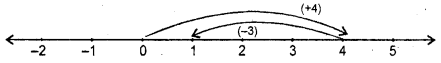First we move 4 steps to the right of 0 reaching 4 and then from this point we move 3 steps to the left. We reach the point 1. Thus, (+4) + (-3) = 1.

Question 3.
Find the solution of the following without using a number line :
(a) (+7) + (-11), (b) (-13) + (+10), (c) (-7) + (+9), (d) (+10) + (-5).
Make five such questions and solve them.
Solution:
(a) (+7) + (-11) = -4,
(b) (-13) + (+10) = -3,
(c) (-7) + (+9) = +2,
(d) (+10) + (-5) = 5.

Question 4.
Find the solution of the following without using a number line :
(i) (+5) + (+3),
(ii) (-6) +(-2),
(iii) (-5)+(+15),
(iv) (-8) + (+5),
(v) (+7) + (+10).
Solution:
(i) (+5) + (+3) = +8,
(ii) (-6) + (-2) = -8,
(iii) (-5) + (+15) = + 10
(iv) (-8) + (+5) = -3,
(v) (+7) + (+10) = 17.